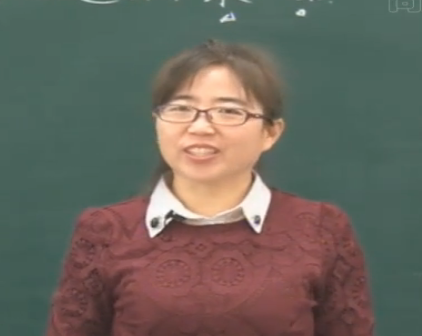尊敬的用户：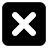三年级数学上册第三章《加与减》节余多少钱—三位数加减混合运算（2）

• 同步课程
• 课后作业
• 同步试卷
• 本节重点
• 主讲老师

318+254-190
=572-190
=382
653+97+203
=750+203
=953
653+97+203
=653+（97+203）
=653+300
=953
427+273-165
=700-165
=535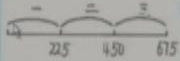225+225=450（元）
450+225=675（元）
675元＞580元

580-225=335（元）
355-225=130（元）
130元＜225元

2.人民剧场楼下有425个座位，楼上比楼下少185个座位，人民剧场一共有多少个座位？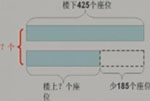425-185=240（个）
240+425=665（个）
425+（425-185）
=425+240
=665（个）

4.王爷爷买了一台电冰箱，先付500元，余下部分每月付260元，几个月能全部付清？
1200-500=700（元）
700-260-260=180（元）
1200-500=700（元）
260+260+260=780（元）

5.冒险乐园上午售出290张票。

（1）水上天地比冒险乐园少售出多少张票？
（2）冒险乐园和卡通世界一共售出多少张票？
（3）请你栽提出一个数学问题，并尝试解答。
1.290+315-475
=605-475
=130（张）
2.290+315+395
=605+395
=1000（张）

940-320-320=300（元）
280+280+280=840（元）

一、填空题

1．填空。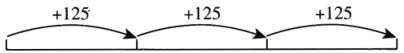472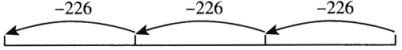700

【参考答案】：597、722、847、22、248、474

二、主观题

1．下面是水站近4个月桶装水的销量。

 月份 6 7 8 9 销量／桶 322 265 375 486
(1)水站在6，7，8三个月里共卖出多少桶水？
(2)哪个月的销量最多？这个月的销量比6，7月销量的总和少多少桶水？
(3)请你试着再提出一个数学问题，并解答。

【参考答案】：(1)322+265+375=962（桶）

(2)322+265-486=101（桶）

(3)问题：水站在7，8，9三个月里共卖出多少桶水？
265+375+486=1126（桶）

2．王叔叔想换一部新手机，但是他现在只有900元。商场允许他支付880元后立刻得到一部新手机（如图），余下的钱需要每个月付280元。王叔叔几个月能全部付清？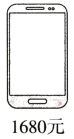【参考答案】：1680-880=800（元）  800-280=520（元）
520-280=240（元）  240元＜280元

560+280=840（元）  840元＞800元

3.计算：998+996+994+992-997-995-993-991【试题解析】：对于这种既有加法又有减法的混合运算，通常可以用“带着符号搬家”的方法，让加法和减法互相结合后，减出比较小的数，再继续计算。

【参考答案】：998+996+994+992-997-995-993-991
=(998-997)+(996-995)+(994-993)+(992-991)
=1+1+1+1
=4